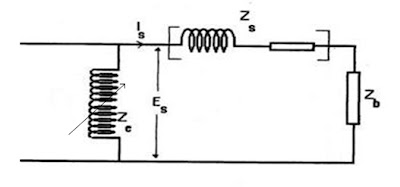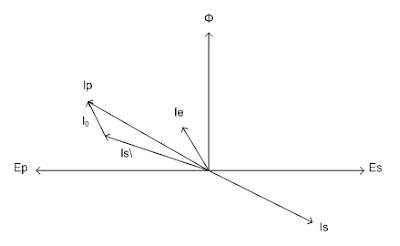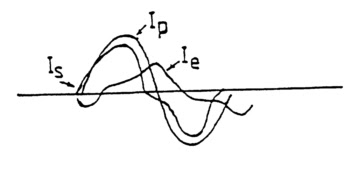### Current Transformer Error

Instrument Transformer and Power Management (P1) Course
Chapter (6) : Current Transformers
6.3.7 Current Transformer Error
The errors associated with current transformers are the current error and the phase error. As shown by the tables the errors for metering and protection current transformers are quite different, those for protection transformers being less exacting, as might be expected.
It follows that a high permeability core material with a low saturation level is most suitable for measuring current transformers , whereas the principal requirement in protection current transformers is a high saturation level, The core materials most suitable for measuring applications are nickel iron alloys as these have by far the lowest exciting ampere turns per unit length of core and the knee point occurs at a relatively low flux density.

Grain orientated silicon steels are used in protection current transformers as although they exhibit a poorer exciting ampere -turns rating, they offer the advantage of a significantly higher knee -point flux density.

6.3.7.1 Ratio error ( current error):
The ratio error is defined as the error in the secondary current due to the incorrect ratio and is expressed as a percentage, by the expression:
{(Kn Is-Ip)/Ip}x100
Where Kn is the nominal ratio ( rated primary current / rated secondary current )
Is is the actual secondary current.
Ip is the actual primary current.
The ratio is considered positive when the actual secondary current of the transformer is larger than the rated current.

6.3.7.2 Phase angle error :
The phase angle error is the angle by which the secondary current vector when reversed, differs in phase from the primary current. This angle is considered as positive if the reversed secondary current vector leads the primary current vector.

On very low burden power factors the phase angle error may be negative only on rare occasions. It is necessary to determine the phase error of a C. T used for relaying. These occasions occur when very low circuit settings i.e. 1% -5% of rated current, are used in relays which are polarized by voltage transformer inputs.

For example :
Sensitive reverse power relays may requires taking phase error into account in order to ensure correct direction operation where very low power factor primary current are involved e.g. 1% - 3% power when certain types of prime movers are been motored e.g. steam turbines and hydro sets.

Considering the excitation impedance (Ze) as a constant. The vectorial relationships between the fundamental frequency currents are typically as shown in Fig. (7). The vectorial difference between Ip and Is is Ie. Im constitutes the current errors and Ip. the component of Ie in quadrature with Is which results in the phase difference.

If Ze were in fact a constant impedance the vectorial error Ie of the diagram would be the composite error but in practice the magnetizing impedance Ze is not constant with the result that the exciting current Ie contains some harmonics of the fundamental frequency which increase its r.m.s value and thus increased the composite error.

This effect is most noticeable in the region approaching saturation of the core when the wave-forms of the primary, secondary and exciting current would be somewhat as shown.Fig. ( 7-a )Fig. ( 7-b )Fig. (7-c)

6.3.7.3 Polarity :
Where current transformers are used with instruments or relays which rely not only on magnitude but also on phase position, polarity is of importance. Polarity gives the relative instantaneous direction of current in the primary and secondary leads. It is indicated by marking one primary and one secondary terminal as shown in the following fig.
By definition, when current is flowing toward the marked H. V primary terminal, it is flowing away from the transformer in the marked secondary lead; this marking corresponds to HI, XI used on single phase power transformer.

Sponsored Articles  :